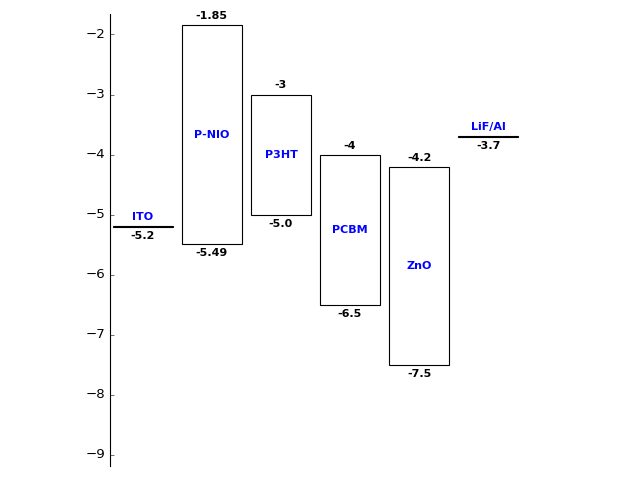The code helps in plotting the energy band diagram of stacks of semiconductors and metals that are commonly used in Solar cell and LED research.

# plasmon360/Energy-Band-diagram

Switch branches/tags
Nothing to show

A tag already exists with the provided branch name. Many Git commands accept both tag and branch names, so creating this branch may cause unexpected behavior. Are you sure you want to create this branch?

## Files

Failed to load latest commit information.
Type
Name
Commit time

# Energy-Band-digram

The code helps in plotting the energy band diagram of stacks of semiconductors and metals that are commonly used in Solar cell and LED research.

Example:

``````from Band_diagram import metal, semiconductor, plot

# # Define the metals and semiconductors
ITO = metal(wf = -5.2, name= 'ITO')
p_nio = semiconductor(cb = -1.85, vb = -5.49, name = 'P-NIO')
p3HT = semiconductor(cb = -3, vb = -5.0, name = 'P3HT')
PCBM = semiconductor(cb = -4, vb = -6.5, name = 'PCBM')
ZnO = semiconductor(cb = -4.2, vb = -7.5, name = 'ZnO')
LiF_Al = metal(wf=-3.7, name = 'LiF/Al')

## Create the stack
stack = [ITO,p_nio,p3HT,PCBM,ZnO,LiF_Al]

## plot the stack
plot(stack, filepath = 'Images/Stack1.png')
``````

will result inThe code helps in plotting the energy band diagram of stacks of semiconductors and metals that are commonly used in Solar cell and LED research.

## Releases

No releases published

## Packages 0

No packages published